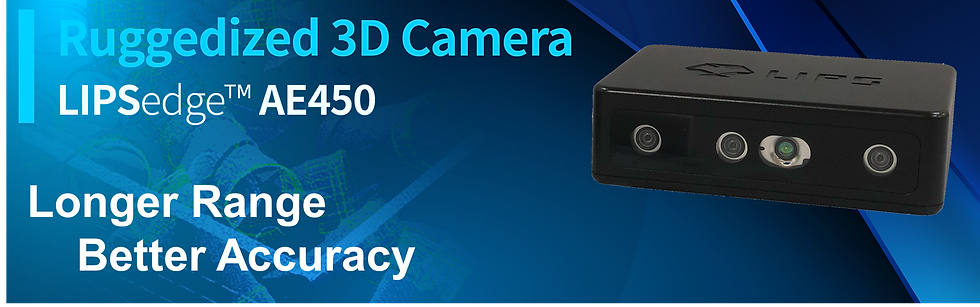top of page
Search
• LIPS

# Deploying LIPSedge AE450™ with OpenCV: A Step-by-Step Guide

Updated: Mar 13Are you looking to integrate OpenCV with LIPSedge AE™450 to utilize depth data? Look no further! In this article, we will show you how to draw a cross line in the center and put depth information on the bottom left of the screen, all while converting a depth frame from LIPSedge AE450 to an OpenCV image matrix.## Step 1: Install OpenCV

Before we dive into the code, we need to make sure OpenCV is installed on our system. You can do this by following the installation guide on the OpenCV website. Make sure to install the correct version based on your system specifications.

• For Ubuntu

`sudo apt install libopencv-dev python3-opencv python3-numpy`
• For Windows

1. Download from OpenCV Releases. Extract the files to where you desired. 2. Add OpenCV directory into PATH environment variable. 3. Install packages for python

`pip3 install numpy opencv`

## Step 2: Write Code

Now that OpenCV is installed, we can begin writing our code. We can modify the code from Hello LIPS AE (C++ / Python) . After creating rs2::pipline, we create a openCV window for given name and a fix size.

• C++

```#include <opencv2/opencv.hpp>
cv::namedWindow(WINDOW_NAME, cv::WINDOW_AUTOSIZE);```
• Python

```import cv2
import numpy as np
WINDOW_NAME = 'depth viewer'
cv2.namedWindow(WINDOW_NAME, cv2.WINDOW_AUTOSIZE)```

Instead of writing a forever loop, OpenCV waitKey funtion allow you to listen to keypressed event. When ESC (key code 27) key is pressed break the loop.

• C++

`while (cv::waitKey(1) != 27) { ... }`
• Python

`while cv2.waitKey(1) != 27:`

After getting depth frame, we apply a colormap convertion and then create a OpenCV image matrix for it. SDK provide 10 different colormaps. You can choose whatever you like by setting index 0 to 9.

• C++

CV_8UC3 is a format for image pixels. 8U means 8 unsigned bit per channel. C3 means 3 channels (Blue, Green and Red).

```cv::Mat image(
cv::Size(w, h),
CV_8UC3,
(void *)colorFrame.get_data(),
cv::Mat::AUTO_STEP
);```
• Python

```colorFrame = rs.colorizer(2).colorize(frame)
colorImage = np.asanyarray(colorFrame.get_data())```

Next, use line function draw a cross line to mark the point we are measuring, which is in the center of frame. Then use putText to display the distance on the bottom left of the screen.

• C++

```cv::line(image, cv::Point(center_x - 15, center_y),
cv::Point(center_x + 15, center_y),
cv::Scalar(0, 0, 255),
2, cv::LINE_8
);
cv::line(image, cv::Point(center_x, center_y - 15),
cv::Point(center_x, center_y + 15),
cv::Scalar(0, 0, 255),
2, cv::LINE_8
);```
• Python

```cv2.line(colorImage, (centerX- 15, centerY), (centerX + 15, centerY), (0, 0, 255))
cv2.line(colorImage, (centerX, centerY- 15), (centerX, centerY + 15), (0, 0, 255))
cv2.putText(colorImage, str(distance), (0, height), 2, 2, (0, 0, 255))```

## Step 3: Full Code Example

Here is the full code example using C++, CMakeLists.txt, and Python for your reference:

• C++

```#include <librealsense2/rs.hpp>
#include <opencv2/opencv.hpp>

#define WINDOW_NAME "Depth Viewer"

int main(int argc, char *argv[])
{
rs2::pipeline pipe;
pipe.start();

cv::namedWindow(WINDOW_NAME, cv::WINDOW_AUTOSIZE);

while (cv::waitKey(1) != 27)
{
rs2::frameset frameset = pipe.wait_for_frames();

// Get depth frame then map to RGB color.
rs2::depth_frame frame = frameset.get_depth_frame();

// Get the frame size.
const int w = frame.as<rs2::video_frame>().get_width();
const int h = frame.as<rs2::video_frame>().get_height();

// Get the coordinate of center position.
const int center_x = w / 2;
const int center_y = h / 2;

// Map depth value to different color.
rs2::colorizer color_map(2);
rs2::frame colorFrame = frame.apply_filter(color_map);
cv::Mat image(cv::Size(w, h), CV_8UC3, (void *)colorFrame.get_data(), cv::Mat::AUTO_STEP);

// Draw a cross line in the center.
cv::line(image, cv::Point(center_x - 15, center_y), cv::Point(center_x + 15, center_y), cv::Scalar(0, 0, 255), 2, cv::LINE_8);
cv::line(image, cv::Point(center_x, center_y - 15), cv::Point(center_x, center_y + 15), cv::Scalar(0, 0, 255), 2, cv::LINE_8);

// Get the depth data at the center and display in the bottom left.
float dist_to_center = frame.get_distance(center_x, center_y);
putText(image, std::to_string(dist_to_center), cv::Point(0, h), 2, 2, cv::Scalar(0, 0, 255));

// Show image in window
imshow(WINDOW_NAME, image);
}

return EXIT_SUCCESS;
}```
• CMakeLists.txt

```cmake_minimum_required(VERSION 3.8)

project(depth-viewer CXX)

set_property(TARGET \${PROJECT_NAME} PROPERTY CXX_STANDARD 11)

find_package(OpenCV REQUIRED COMPONENTS core highgui)
• Python

```import pyrealsense2 as rs
import cv2
import numpy as np

# Create RealSense API object
pipe = rs.pipeline()
# Start video streaming
pipe.start()

# Create window
WINDOW_NAME = 'depth viewer'
cv2.namedWindow(WINDOW_NAME, cv2.WINDOW_AUTOSIZE)

try:
while cv2.waitKey(1) != 27:
# Wait Until frames are available
frameset = pipe.wait_for_frames()

# Get a depth frame image.
frame = frameset.get_depth_frame()

# Get frame size.
width = frame.get_width()
height = frame.get_height()

# Get the coordinate of center position.
centerX = int(width / 2)
centerY = int(height / 2)

# Map depth value to different colors.
colorFrame = rs.colorizer(2).colorize(frame)
colorImage = np.asanyarray(colorFrame.get_data())

# Draw a cross line in the center
cv2.line(colorImage, (centerX - 15, centerY), (centerX + 15, centerY), (0, 0, 255))
cv2.line(colorImage, (centerX, centerY - 15), (centerX, centerY + 15), (0, 0, 255))

# Get distance from the camera to the center of this frame
distance = frame.get_distance(int(width / 2), int(height / 2))
cv2.putText(colorImage, str(distance), (0, height), 2, 2, (0, 0, 255))

# Show image in window
cv2.imshow(WINDOW_NAME, colorImage)
finally:
pipe.stop()```

## Conclusion

In this article, we have shown you how to deploy LIPSedge AE™450 with OpenCV to utilize depth data. By following the step-by-step guide and using the provided code, you can easily convert a depth frame from LIPSedge AE450 to an OpenCV image matrix and display the depth information on the screen. If you have any questions, please post them to LIPS Forum.